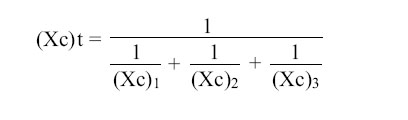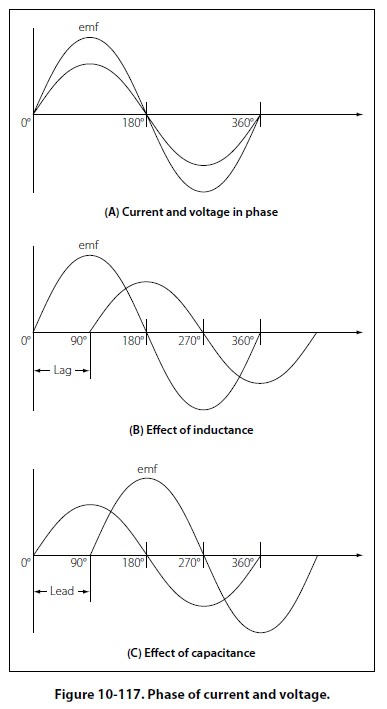Capacitive Reactances in Series and in Parallel When capacitors are connected in series, the total reactance is equal to the sum of the individual reactances. Thus, Xct = (Xc)1 + (Xc)2 The total reactance of capacitors connected in parallel is found in the same way total resistance is computed in a parallel circuit:Phase of Current and Voltage in Reactive Circuits Unlike a purely resistive circuit, the capacitive and inductive reactance has a significant effect on the phase relationship between the applied AC voltage and the corresponding current in the circuit. In review, when current and voltage pass through zero and reach maximum value at the same time, the current and voltage are said to be in phase. [Figure 10-117A] If the current and voltage pass through zero and reach the maximum values at different times, the current and voltage are said to be out of phase. In a circuit containing only inductance, the current reaches a maximum value later than the voltage, lagging the voltage by 90°, or one-fourth cycle. [Figure 10-117B]In a circuit containing only capacitance, the current reaches its maximum value ahead of the voltage and the current leads the voltage by 90°, or one-fourth cycle. [Figure 10-117C] The amount the current lags or leads the voltage in a circuit depends on the relative amounts of resistance, inductance, and capacitance in the circuit.
 ©AvStop Online Magazine                                                                                                                                                      Contact Us              Return To Books